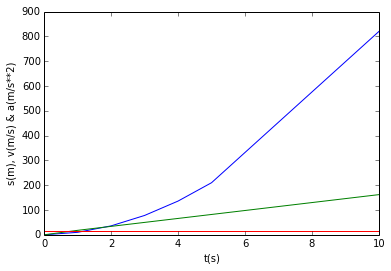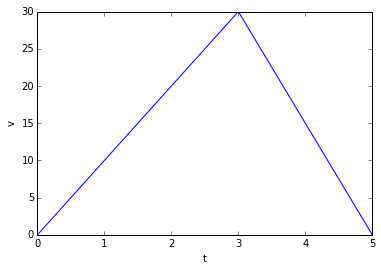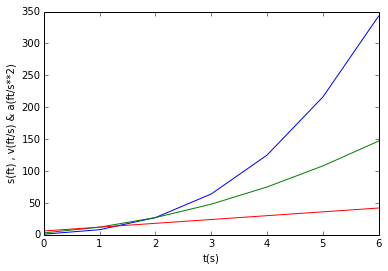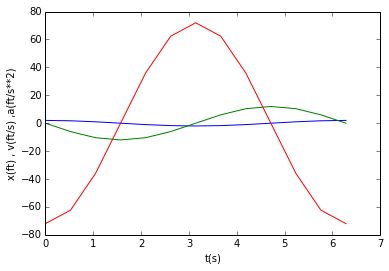Chapter 12: Kinematics of a Particle¶

Ex 12.12-1, Page No 200¶

In :
import math

# Initilization of variables
t=4 #seconds

# Calculations
#Displacement
x=3*t**3+t+2 #ft
# Velocity
v=9*t**2+1 # ft/s
# Acceleration
a=18*t # ft/s**2

# Result
print'The dipalacemnt is',round(x),"ft"
print'The velocity is ',round(v),"ft/s"
print'The acceleration is ',round(a),"ft/s**2"
The dipalacemnt is 198.0 ft
The velocity is  145.0 ft/s
The acceleration is  72.0 ft/s**2

Example 12.12-2, Page No 201¶

In :
import math

# Initilization of variables
t1=4 #s
t2=5 #s

# Calculation
v1=9*t1**2+1 # ft/s
v2=9*t2**2+1 # ft/s
a=(v2-v1)/(t2-t1) # m/s**2

# Result
print'The acceleration during fifth second is',round(a),"ft/s**2"
The acceleration during fifth second is 81.0 ft/s**2

Example 12.12-3, Page No 201¶

In :
import math
%matplotlib inline

#Defining Matrices
t=[0,1,2,3,4,5,10] #s
# equation for s is s=8*t**2+2*t, Thus the different values of s corresponding to t is:
#Displacement matrix
s=[0,10,36,78,136,210,820]
# Eqn for v is v=16*t+2,Thus the different values of v corresponding to t is:
#Velocity Matrix
v=[0,18,34,50,66,82,162]
# Eqn for a is a=16, Thus the different values of a corresponding to t is:
#Acceleration Matrix
a=[16,16,16,16,16,16,16]
#Plotting the curves
#S-T curve
plot(t,s)
plot(t,v)
plot(t,a)
xlabel('t(s)')
ylabel('s(m), v(m/s) & a(m/s**2)')

#Result
print'The graphs are the solutions'
print'blue line is for "s" vs "t" '
print'green line is for "v" vs "t" '
print'red line is for "a" vs "t" '
# All the 3 graphs have been combined into a single graph
The graphs are the solutions
blue line is for "s" vs "t"
green line is for "v" vs "t"
red line is for "a" vs "t"Example No 12.12-4, Page No 202¶

In :
import math

#Initilization of variables
v_o=0 #ft/s
v_f=88#ft/s
t=28 #s

#Calculations
k=(v_f-v_o)*t**-1 #ft/s**2
s=((v_f-v_o)/2)*t #ft

#Result
print'The value of constant k is',round(k,2),"ft/s**2"
print'The displacement is ',round(s),"ft"
#Decimal accuracy causes discrepancy in answers
The value of constant k is 3.14 ft/s**2
The displacement is  1232.0 ft

Example 12.12-5, Page No 202¶

In :
import math
%matplotlib inline

#Initilization of variables
v_o=0 #ft/s
v_f1=30 #ft/s
v_f2=0 #ft/s
t1=3 #s
t2=2 #s

#Calculations
#Plotting the v-t curve
#Velocity matrix
v=[v_o,v_f1,v_f2]
#Time matrix
t=[0,3,5]
plot(t,v)
xlabel('t')
ylabel('v')
#Part "b"
#Acceleration at 3s
a1=(v_f1-v_o)/t1 #ft/s**2
#Acceleration at 5s
a2=(v_f2-v_f1)/t2 #ft/s**2
#Part "c"
s=(v_f1*t1*0.5)+(v_f1*t2*0.5) #ft
#Part "d"
#Simplfying the equation we get
#7.5t**2-30t+5=0
a=7.5
b=-30
c=5
q=sqrt(b**2-4*a*c)
x1=(-b+q)/(2*a)
x2=(-b-q)/(2*a)
#As x1 is greater than 2 it does not hold as a solution
t=x2 #s
#Hence total time is
T=t1+t #s

#Result
print'The graph is the solution for part a'
print'The acceleration at 3rd second is',round(a1),"ft/s**2"
print'The acceleration at 5th second is',round(a2),"ft/s**2"
print'The displacement is',round(s),"ft"
print'The total time is',round(T,3),"s"
The graph is the solution for part a
The acceleration at 3rd second is 10.0 ft/s**2
The acceleration at 5th second is -15.0 ft/s**2
The displacement is 75.0 ft
The total time is 3.174 sExample No 12.12-6, Page No 203¶

In :
import math

#Initilization of variables
v_o=2 #m/s
y_o=120 #m
g=9.8 #m/s**2

#Calculations
#Solve  using ground as datum
y=0
#Simplfying the equation
a=4.9
b=-2
c=-120
q=sqrt(b**2-4*a*c)
x1=(-b+q)/(2*a) #s
x2=(-b-q)/(2*a) #s

#Result
print'The time required is',round(x1,2),"s"
#As x2 is negative and negative time does not make any physical sense
The time required is 5.16 s

Example 12.12-7, Page No 204¶

In :
import math

#Initilization of variables
Vo1=80 #ft/s
Vo2=60 #ft/s
g=32.2 #ft/s**2

#Calculations
#Simplfying by equating the two times
t=(-(Vo2*2)-(g*0.5*4))/(Vo1-Vo2-(g*0.5*4)) #s
#Substituting this t in s we get
s=(Vo1*t)-(0.5*g*t*t) #ft

#Result
print'The time obtained is',round(t,2),"s"
print'and the ball meets at',round(s,1),"ft"
The time obtained is 4.15 s
and the ball meets at 54.5 ft

Example 12.12-8, Page No 204¶

In :
import math

#Initilization of variables
# as theta=40 degrees
costheta=0.77
tantheta=0.83
x=100 #ft
ay=32.2 #ft/s**2

#Calculations
#Simplfying the equation
t=((tantheta*x)*(ay/2)**-1)**0.5 #s
#Velocity calculations
Vo=100*(costheta*t)**-1 #ft/s

#Result

print'The initial speed should be',round(Vo,1),"ft/s"
The initial speed should be 57.2 ft/s

Example 12.12-9, Page No 204¶

In :
import math
%matplotlib inline

#Initilization of variables
t=[0,1,2,3,4,5,6] #s
#Solving the Differential Equations we obtain
# Eqn for s is s=(t+1)**3,Thus the different values of s corresponding to t is:
#Displacement matrix
s=[1,8,27,64,125,216,343]
# Eqn for v is v=3*(t+1)**2, Thus the different values of v corresponding to t is:
# Velocity matrix
v=[3,12,27,48,75,108,147]
# Eqn for a is a=6*(t+1),Thus the different values of a corresponding to t is:
# Acceleration matrix
a=[6,12,18,24,30,36,42]
#Plotting
plot(t,s)
plot(t,v)
plot(t,a)
xlabel('t(s)')
ylabel('s(ft) , v(ft/s) & a(ft/s**2)')

#Result
print'The result are the plots that have been generated'
print'blue line is for "s" vs "t"'
print'green line is for "v" vs "t"'
print'red line is for "a" vs "t"'
# All the graphs have been plotted on a single graph
The result are the plots that have been generated
blue line is for "s" vs "t"
green line is for "v" vs "t"
red line is for "a" vs "t"Example 12.12-10, Page No 205¶

In :
import math

#Initilization of variables
t=3 #s

#Calculations
#After solving the differential equation
s=(3**-1)*(t+2)**3 #ft
v=(t+2)**2 #ft/s
a=2*(t+2) #ft/s**2

#Result
print'The displacement at t=3s is',round(s,1),"ft"
print'The velocity at t=3s is',round(v),"ft/s"
print'The acceleration at t=3s is',round(a),"ft/s**2"
The displacement at t=3s is 41.7 ft
The velocity at t=3s is 25.0 ft/s
The acceleration at t=3s is 10.0 ft/s**2

Example 12.12-12, Page No 206¶

In :
import math

#Initilization of variables
#Calling upward direction positive
xdot1=6 #ft/s
xdot3=3 #ft/s
xdoubledot=2 #ft/s**2
xdoubledot3=-4 #ft/s**2

#Calculations
xdot=-xdot1 #ft/s
xdot2=2*xdot-xdot3 #ft/s
xdoubledot2=2*xdoubledot-xdoubledot3 #ft/s**2

#Result
print'The value of velocity is',round(xdot2,3),"ft/s (down)"
print'The value of acceleration is',round(xdoubledot2,3),"ft/s**2"
The value of velocity is -15.0 ft/s (down)
The value of acceleration is 8.0 ft/s**2

Example 12.12-16, Page No 207¶

In :
import math

#Initilization of variables
t=4 #s

#Calculations
#Part (a)
x=t**3 #in
y=-2*t**2 #in
z=2*t #in
#Part (b)
#Theory question
#Part(c)
#Unit vector calculation
m=(4**2+1**1+(-3)**2)**0.5
e_l=[4*m**-1,m**-1,-3*m**-1]
v=[3*t**2,-4*t,2] #in/s
#Projection of v on n at t=4s
dot=[v*e_l,v*e_l,v*e_l]
#dot=v.*e_l #in/s
a=dot+dot+dot #in/s

#Result
print'The co-ordinates of position are x=',round(x),"in ,",round(y),"in and ",round(z),"in respectively"
print'The projection of v on n at t=4s is',round(a,1),"in/s"
The co-ordinates of position are x= 64.0 in , -32.0 in and  8.0 in respectively
The projection of v on n at t=4s is 33.3 in/s

Example 12.12-17, Page No 208¶

In :
import math

#Initilization of variables

#Calculations
#Method (a)
t=(theta)**0.5 #s
r=2*theta
rdot=4*t
#Velocity calculations
v=((rdot)**2+x**2)**0.5 #ft/s
#Theta calculations
thetax=30+arctan(rdot/x)*(180/pi) #degrees
#Method (b)
x=2*theta*cos(theta) #ft
y=2*theta*sin(theta) #ft
xdot=4*t*((cos(t**2)))+2*t**2*(-sin(t**2))*(2*t) #ft/s
ydot=4*t**2*sin(t**2)+2*t**2*cos(t**2)*2*t #ft/s
V=(xdot**2+ydot**2)**0.5 #ft/s
Thetax=arctan(ydot/-xdot)*(180/pi) #degrees

#Result
print'By both the methods we obtain v and thetax as:'
print'Method 1'
print'v=',round(v,2),"ft/s",'and thetax=',round(thetax,1),"degrees"
print'Method 2'
print'V=',round(v,2),"ft/s",'and Thetax=',round(Thetax,1),"degrees"
# The answer may wary due to decimal point accuracy
By both the methods we obtain v and thetax as:
Method 1
v= 5.93 ft/s and thetax= 73.7 degrees
Method 2
V= 5.93 ft/s and Thetax= 73.9 degrees

Example 12.12-18, Page No 209¶

In :
import math

#Initilization of variables

#Calculations
t=sqrt(theta) #s
r=2*t**2
rdot=4*t
rdoubledot=4
a=sqrt(ax**2+ay**2) # fr/s**2
thetax=30+arctan(ax/ay)*(180/pi) #degrees
#Solving by cartesian co-ordinate system yields same solution

#Result
print'The value of acceleration is',round(a,1),"ft/s**2"
print'The value of thetax is',round(thetax,1),"degrees"
#Decimal accuracy causes discrepancy in answers
# The ans for thetax is incorrcet in textbook
The value of acceleration is 21.4 ft/s**2
The value of thetax is 18.2 degrees

Example 12.12-21, Page No 211¶

In :
import math

#Initilization of variables
Va=5 #ft/s
# as theta=70 degrees
sintheta=0.94
costheta=0.34
l=6.24 #ft

#Calculations
Vb=(-costheta/sintheta)*Va #ft/s

#Result
print'The value of Vb is',round(Vb,2),"ft/s"
The value of Vb is -1.81 ft/s

Example 12.25-25, Page No 214¶

In :
import math
import numpy as np
%matplotlib inline

#Initilization of variables
theta=linspace(0,360,13)

#Calculations
#Defining everything in terms of matrices
t=(theta*pi)/(180) #s converting degrees to radians
costheta=cos(t)
sintheta=sin(t)
x=2*costheta #ft
v=-12*sintheta #ft/s
a=-72*costheta #ft/s**2

#Plotting
# 1
plot(t,x)
# 2
plot(t,v)
# 3
plot(t,a)
xlabel('t(s)')
ylabel('x(ft) , v(ft/s) ,a(ft/s**2)')

#Result
print'The results are the plots'
print'The curve in blue colour represents t vs x'
print'The curve in green represents t vs v'
print'The curve in red represents t vs a'
# All the 3 curves have been plotted in the same graph.
The results are the plots
The curve in blue colour represents t vs x
The curve in green represents t vs v
The curve in red represents t vs aExample 12.12-26, Page No 215¶

In :
import math

#Initilization of variables
d=1.2 #m
w0=0 #rpm
w=2000 #rpm
t=20 #s

#Calculations
alpha=(w-w0)/t

#Result
The angular acceleration is 10.5 radians/s**2

Example 12.12-27, Page No 216¶

In :
import math

#Initilization of variables
t=20 #s

#Calculations
theta_rev=round(theta/(2*pi)) #revolutions rounding off

#Result
print'The flywheel makes',round(theta_rev),"revolutions"
The flywheel makes 333.0 revolutions

Example 12.12-28, Page No 216¶

In :
import math

#Initilization of variables
t=0.6 #s
r=0.6 #m

#Calculations
v=r*w #m/s
a_t=r*alpha #m/s**2
a_n=r*w*w #m/s**2
a=sqrt(a_t**2+a_n**2) #m/s**2
phi=arctan(a_t/a_n)*(180/pi) #degrees

#result
print'The tangential velocity is',round(v,2),"m/s"
print'The acceleration is',round(a,1),"m/s**2"
print'and the angle is',round(phi,1),"degrees"
The tangential velocity is 3.78 m/s
The acceleration is 24.6 m/s**2
and the angle is 14.8 degrees

Example 12.12-29, Page No 216¶

In :
import math

#Initilization of variables
l=4 #ft
wb=40 #rpm
we=60 #rpm

#Calculations
r=l/2 #ft
vb=r*((wb*2*pi)/60) #ft/s
ve=r*((we*2*pi)/60) # ft/s

#Result
print'The linear speeds are:'
print'vb=',round(vb,2),"ft/s"
print'and ve=',round(ve,1),"ft/s"
The linear speeds are:
vb= 8.38 ft/s
and ve= 12.6 ft/s

Example 12.12-30, Page No 217¶

In :
import math

#Initilization of variables
wb=40 #rpm
we=60 #rpm
t1=5 #s using different symbol to avoid conflict in decleration
t=2 #s
#Calculations

#Components of acceleration are
a_t=r*alpha #ft/s**2
a_n=r*w**2 #ft/s**2

#result
print'The tangential acceleration is',round(a_t,3),"ft/s**2"
print'The normal acceleration is',round(a_n,1),"ft/s**2"
The tangential acceleration is 0.838 ft/s**2
The normal acceleration is 50.5 ft/s**2

Example 12.12-31, Page No 217¶

In :
import math

#Initilization of variables
d=200 #mm
w0=(800*2*pi)/60 #rpm
w=0 #rpm
t=600 #s

#Calculations

#result
The angular acceleration is -0.14 radian/s**2

Example 12.12-32, Page No 217¶

In :
import math

#Initilization of variables
#The symbols used here differ from the textbook solution to avoid conflict
t1=0 #s
t2=0.5 #s
t3=2.5 #s
t4=3**-1 #s
w=200 #rpm
w0=0 #rpm

#Calculations
theta1=0.5*(w0+(w*60**-1))*t2 #rev
theta2=(w*60**-1)*(t3-t2) #rev
theta3=(2**-1)*((w*60**-1)+w0)*t4 #rev here the values of w and w0 are interchanged but essentially the value comes out to be the same hence the decleration has not been changed
theta=theta1+theta2+theta3 #rev

#Result
print'The wheel undergoes',round(theta,2),"revolutions"
The wheel undergoes 8.06 revolutions

Example 12.12-34, Page No 218¶

In :
import math

#Initilization of variables
t=1 #s
r=4 #m

#Calculations
s=t**3+3 #m
Vx=-4*sin(theta)*dtheta_dt #m/s
Vy=4*cos(theta)*dtheta_dt #m/s
V=(Vx**2+Vy**2)**0.5 #m/s

#Result
print'The components of  velocity are:'
print'Vx=',round(Vx,2),"m/s"
print'Vy=',round(Vy,2),"m/s"
print'V=',round(V),"m/s"
The components of  velocity are:
Vx= -2.52 m/s
Vy= 1.62 m/s
V= 3.0 m/s

Example 12.12-35, Page No 218¶

In :
import math

#Initilization of variables
t=1 #s

#Calculations
ax=-4*cos(theta)*dtheta_dt**2-(4*sin(theta)*acc) #m/s**2 (to left)
ay=-4*sin(theta)*dtheta_dt**2+(4*cos(theta)*acc) #m/s**2 (up)
a=sqrt(ax**2+ay**2) #m/s**2

#result
print'The acceleration is',round(a,2),"m/s**2"
The acceleration is 6.41 m/s**2

Example 12.12-36, Page No 218¶

In :
import math

#Initilization of variables
t=2 #s

#Calculations
#Velocity
vx=8*t-3 #ft/s
vy=3*t**2 #ft/s
v=sqrt(vx**2+vy**2) #ft/s
theta_x=arctan(vy*vx**-1)*(180/pi) #degrees
#Acceleration
ax=8 #ft/s**2
ay=6*t #ft/s**2
a=sqrt(ax**2+ay**2) #ft/s**2
phi_x=arctan(ay*ax**-1)*(180/pi) #degrees

#Result
print'The velocity is',round(v,1),"ft/s"
print'and the angle is',round(theta_x,1),"degrees"
print'The acceleration is',round(a,1),"ft/s**2"
print'and the angle it makes is',round(phi_x,1),"degrees"
The velocity is 17.7 ft/s
and the angle is 42.7 degrees
The acceleration is 14.4 ft/s**2
and the angle it makes is 56.3 degrees

Example 12.12-37, Page No 219¶

In :
import math

#Initilization of variables
V_ao=29.3 #ft/s
OA=50 #ft
theta=45 #degrees
OB=50*sqrt(2) #ft

#Calculations
V_bo=V_ao*cos(theta) #ft/s

#Result
print'The angular velocity with respect to the observer is',round(w_ao,3),"rad/s"
print' The angular velocity after moving 50ft is',round(w_bo,3),"rad/s"
# The answer for w_bo is incorrect in textbook
The angular velocity with respect to the observer is 0.586 rad/s
The angular velocity after moving 50ft is 0.218 rad/s

Example 12.12-38, Page No 219¶

In :
import math

#Initiliztaion of variables
# as theta=30 degrees
costheta=sqrt(3)*2**-1
tantheta=sqrt(3)**-1
r=[100*tantheta*(180/pi),100] #ft
v=17.6 #ft/s

#Calculations
v_1=100*costheta**-1*costheta**-1

#result
The angular velocity is 0.132 rad/s clockwise

Example 12.12-39, Page No 220¶

In :
import math

#Initilization of variables
t=2 #s

#Calculations
Vx=20*t+5 #m/s
Vy=t**2-20 #m/s
#As indefinite integral is not possible
x=10*t**2+5*t+5 #m
y=0.5*t**2-20*t-15 #m
ax=20 #m/s**2
ay=2*t #m/s**2

#Result
print'The displacement components are x=',round(x),"m",'and y=',round(y),"m."
print'The velocity components are: Vx=',round(Vx),"m/s",'and Vy=',round(Vy),"m/s"
print'The acceleration components are: ax=',round(ax),"m/s**2",'and ay=',round(ay),"m/s**2"
The displacement components are x= 55.0 m and y= -53.0 m.
The velocity components are: Vx= 45.0 m/s and Vy= -16.0 m/s
The acceleration components are: ax= 20.0 m/s**2 and ay= 4.0 m/s**2

Example 12.12-40, Page No 221¶

In :
import math

#Initilization of variables
d=0.1 #m
v=20 #m/s
a_g=6 #m/s**2
d2=0.150 #m

#Calculations
r=d/2 #m
vb=d2*0.5*w #m/s
a_n=d2*0.5*w*w #m/s**2 normal acceleration
a=sqrt(a_t**2+a_n**2) #m/s**2 linear acceleration

#Result
print'The linear velocity is',round(vb),"m/s"
print'The acceleration is',round(a),"m/s**2"
The linear velocity is 30.0 m/s
The acceleration is 12000.0 m/s**2

Example 12.12-41, Page No 221¶

In :
import math

#Initilization of variables
# as theta=40 degrees
sintheta=0.64
costheta=0.77
tantheta=0.83
x=100 #ft
ax=0 #ft/s**2
ay=-32.2 #ft/s**2

#Calculations
#vox=vocos40....(1)
#voy=vox*t-1/2(32.2)t^2...(2)
#Simplyfying eq (1) and eq(2)
t_f=((x*tantheta)/(0.5*(-ay)))**0.5 #s time of flight
Vo=x/(costheta*t_f) #ft/s
#As the max height occurs at half wat through the flight
t=t_f/2 #s
ymax=Vo*sintheta*t+(0.5*ay*t*t) #ft the formula has positive sign as ay is defined negative

#result
print'The max height the ball will reach is',round(ymax,1),"ft"

# The ans in textbook is incorrect
The max height the ball will reach is 20.8 ft